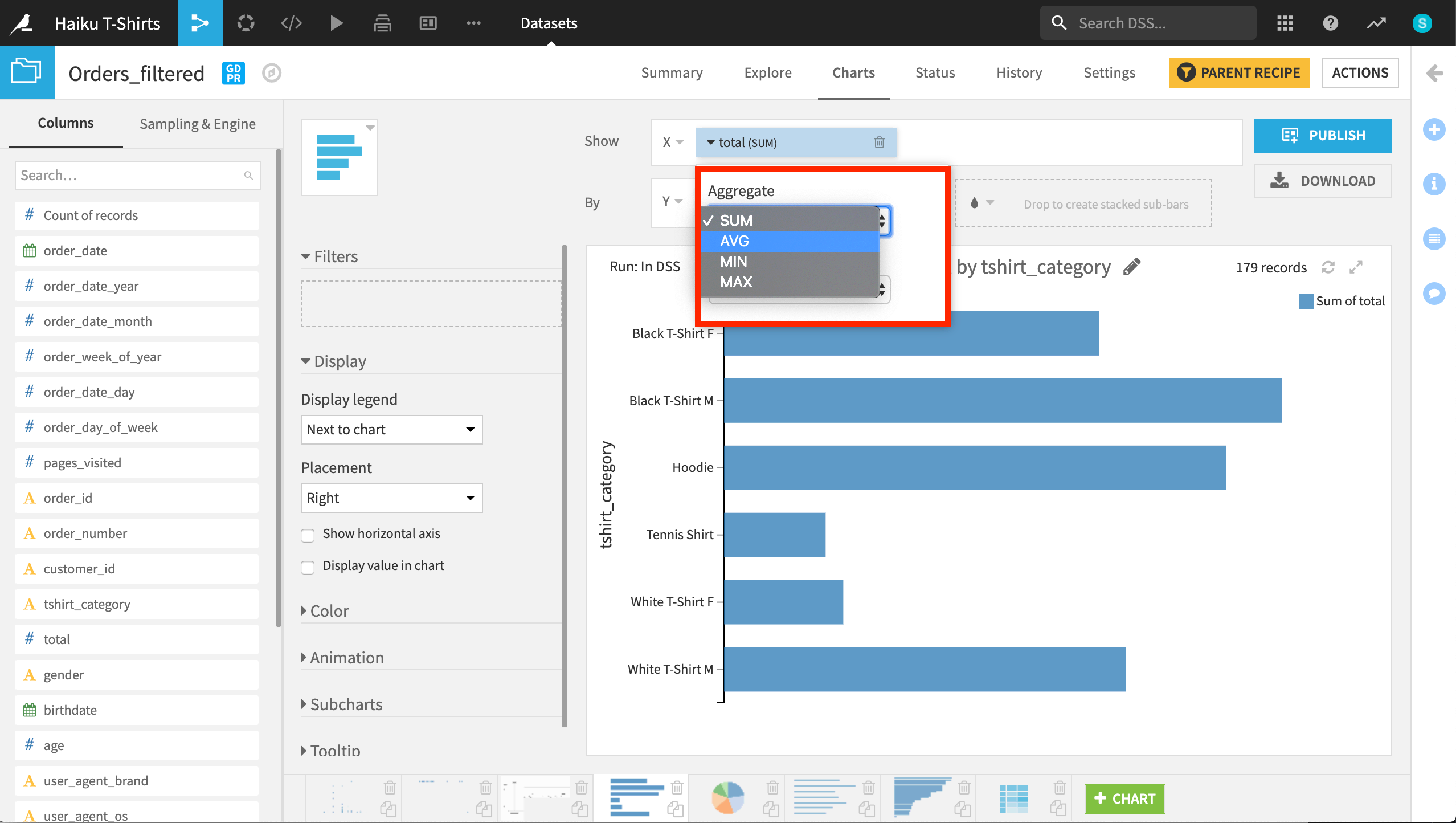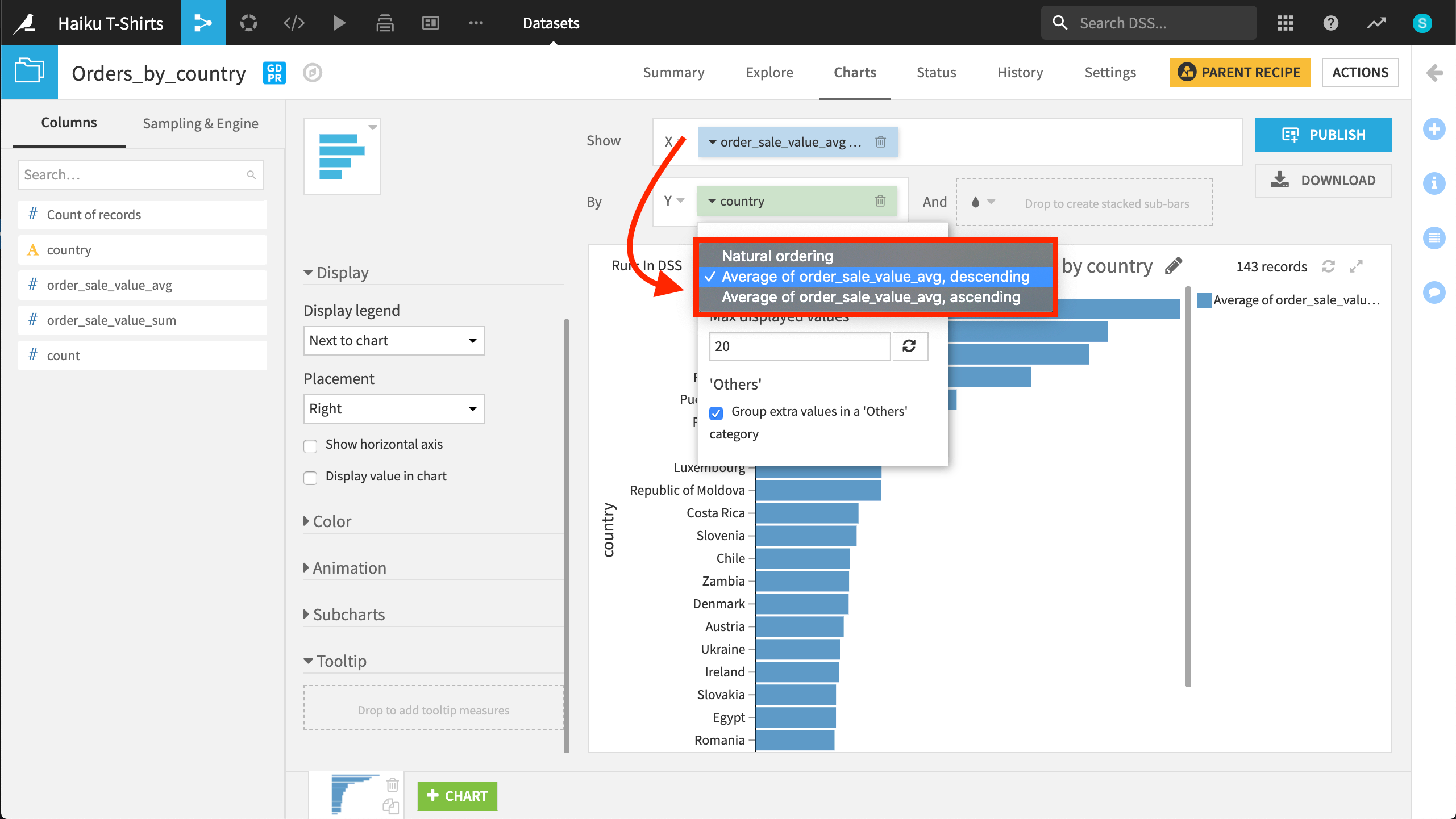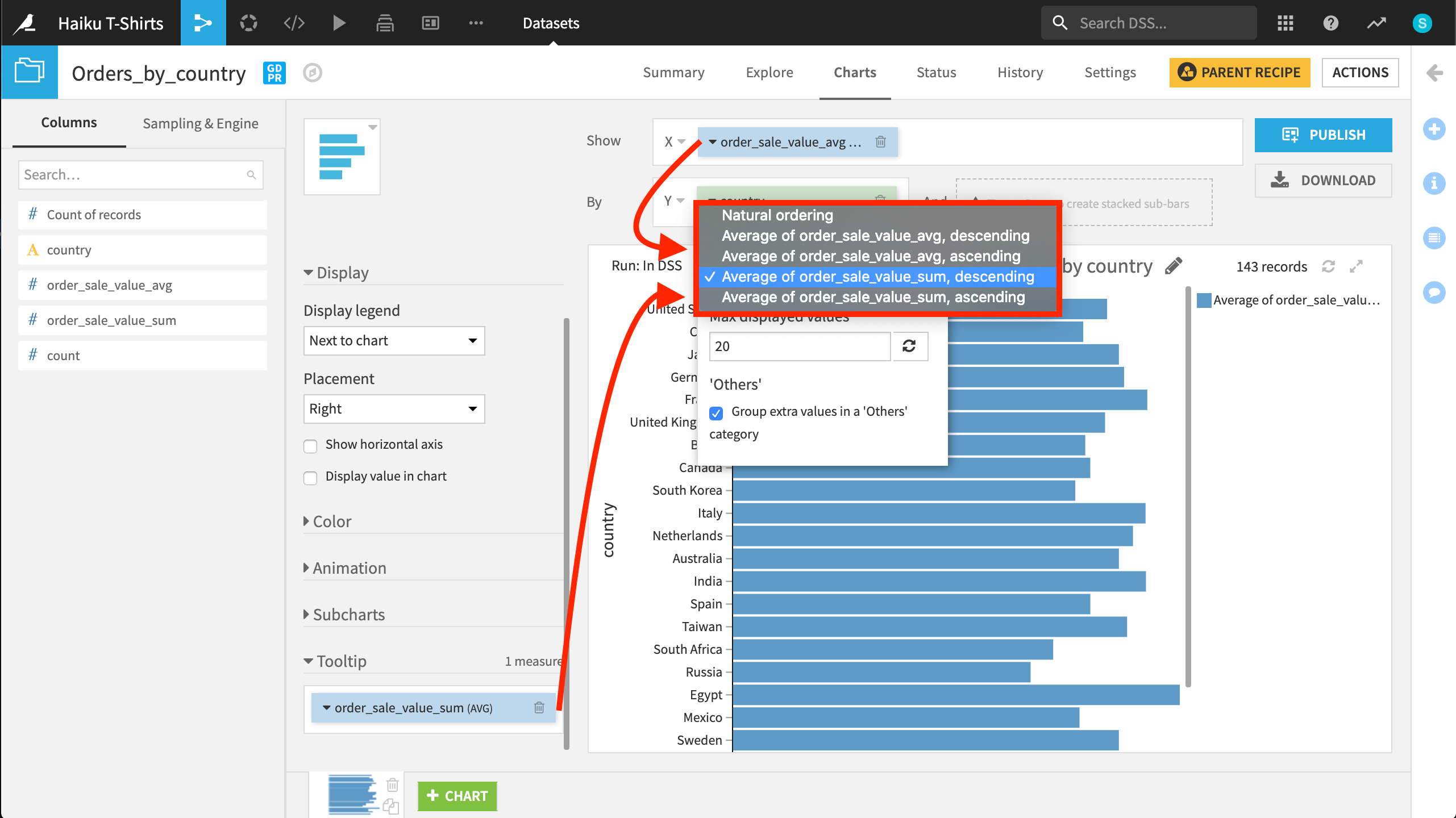# Charts¶

With 25 built-in chart formats –– from histograms, to boxplots, to maps –– drag and drop your data to automatically compute charts on your existing infrastructure (SQL or Impala/Hadoop) for optimal performance.

## FAQ | How do I display non-aggregated metrics in charts?¶

Some types of data visualizations plot raw (or non-aggregated) values, while other visualizations calculate some kind of aggregation. A scatter plot, for example, plots raw values, while a histogram plots bin counts.

The type of chart determines whether displaying raw values is possible.

### Native charts¶

The drag-and-drop interface for creating charts in Dataiku has five native chart types:

• Basics

• Pivot tables

• Scatters

• Maps (geographic)

• Others (such as boxplot or 2D distribution)

Dataiku natively supports using non-aggregated values in scatter plots. For creating other types of charts, it is possible to plot non-aggregated values when grouping by a dimension with unique values. This is often most useful when the data is already in a summarized format.

In cases where the grouping variable does not have unique values, be sure to select the correct aggregation through the interface. For example, should Dataiku sum the values? Take an average? Or plot the minimum or maximum value in the group?If “total” is the amount spent in dollars, should Dataiku plot the sum or average for each type of t-shirt category?

## FAQ | How do I sort on a measure not displayed in charts?¶

When creating a chart (for example a column chart), you can sort the dimension (i.e. the columns) either:

• By its “natural ordering” (i.e. alphabetical or numerical ordering)

• Or by the ordering of a “measure”, i.e. the result of an aggregation. For example, the count of records, the average of the age, …

However, this only allows you to sort based on measures that are “displayed” in the chart. There are some cases where you may want to sort on another measure.

For example, if you are graphing the average sale amount per country, you may want to order your countries, not by the average sale amount but by the total sale amount.

To be able to do that, drag the measure you want to sort on to the “Tooltip” section of the Chart designer. This will put this measure in the tooltip, and allow you to sort based on it.

For example, in the chart below, you can sort values on three measures: natural ordering and the ascending or descending average of the X variable.However, in the same chart below, a Tooltip variable has been added. Now, you can sort the values in the Chart on five measures: the previous three and the ascending and descending average of the new Tooltip variable.